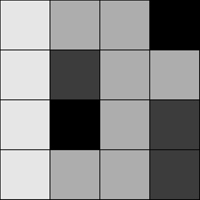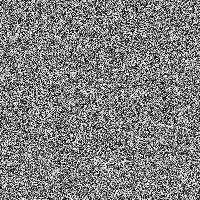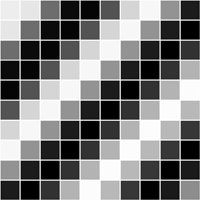# Two-Dimensional Lists

 This tutorial is for Processing's Python Mode. If you see any errors or have comments, please let us know. This tutorial is adapted from the book, Learning Processing, by Daniel Shiffman, published by Morgan Kaufmann Publishers, Copyright © 2008 Elsevier Inc. All rights reserved.   A list keeps track of multiple pieces of information in linear order, or a single dimension. However, the data associated with certain systems (a digital image, a board game, etc.) lives in two dimensions. To visualize this data, we need a multi-dimensional data structure, that is, a multi-dimensional list. A two-dimensional list is really nothing more than an list of lists (a three-dimensional list is a list of lists of lists). Think of your dinner. You could have a one-dimensional list of everything you eat: (lettuce, tomatoes, salad dressing, steak, mashed potatoes, string beans, cake, ice cream, coffee) Or you could have a two-dimensional list of three courses, each containing three things you eat: (lettuce, tomatoes, salad dressing) and (steak, mashed potatoes, string beans) and (cake, ice cream, coffee) In the case of a list, our old-fashioned one-dimensional list looks like this: ``` myList = [0,1,2,3] ``` And a two-dimensional list looks like this: ``` myList = [ [0,1,2,3], [3,2,1,0], [3,5,6,1], [3,8,3,4] ] ``` For our purposes, it is better to think of the two-dimensional list as a matrix. A matrix can be thought of as a grid of numbers, arranged in rows and columns, kind of like a bingo board. We might write the two-dimensional list out as follows to illustrate this point: ``` myList = [ [0, 1, 2, 3], [3, 2, 1, 0], [3, 5, 6, 1], [3, 8, 3, 4] ] ``` We can use this type of data structure to encode information about an image. For example, the following grayscale image could be represented by the following list:``` myList = [ [236, 189, 189, 0], [236, 80, 189, 189], [236, 0, 189, 80], [236, 189, 189, 80] ] ``` To walk through every element of a one-dimensional list, we use a for loop, that is: ``` myList = [0,1,2,3,4,5,6,7,8,9]; for index in len(myList): myList[index] = 0 # Set element at "index" to 0. ``` For a two-dimensional list, in order to reference every element, we must use two nested loops. This gives us a counter variable for every column and every row in the matrix. ``` myList= [ [0, 1, 2] [3, 4, 5] [6, 7, 8] ] # Two nested loops allow us to visit every spot in a 2D list. # For every column i, visit every row j. for i in len(myList): for j in len(myList): myList[i][j] = 0 ``` For example, we might write a program using a two-dimensional list to draw a grayscale image.``` # Example: 2D List def setup(): size(200,200) nRows = height nCols = width myList = make2dList(nRows, nCols) drawPoints(myList) def make2dList(nRows, nCols): newList = [] for row in xrange(nRows): # give each new row an empty list newList.append([]) for col in xrange(nCols): # Make every column in every row a random int from 0 to 255 newList[row].append(int(random(255))) return newList def drawPoints(pointList): for y in xrange(len(pointList)): for x in xrange(len(pointList)): stroke(pointList[y][x]) rect(x,y,10,10) ``` A two-dimensional list can also be used to store objects, which is especially convenient for programming sketches that involve some sort of "grid" or "board." The following example displays a grid of Cell objects stored in a two-dimensional list. Each cell is a rectangle whose brightness oscillates from 0-255 with a sine function.``` Example: 2D Array of Objects # Number of columns and rows in the grid nCols = 10; nRows = 10; def setup(): global nCols, nRows, grid size(200,200) grid = makeGrid() for i in xrange(nCols): for j in xrange(nRows): # Initialize each object grid[i][j] = Cell(i*20,j*20,20,20,i+j) def draw(): global nCols, nRows, grid background(0) # The counter variables i and j are also the column and row numbers and # are used as arguments to the constructor for each object in the grid. for i in xrange(nCols): for j in xrange(nRows): # Oscillate and display each object grid[i][j].oscillate() grid[i][j].display() # Creates a 2D List of 0's, nCols x nRows large def makeGrid(): global nCols, nRows grid = [] for i in xrange(nCols): # Create an empty list for each row grid.append([]) for j in xrange(nRows): # Pad each column in each row with a 0 grid[i].append(0) return grid # A Cell object class Cell(): # A cell object knows about its location in the grid # it also knows of its size with the variables x,y,w,h. def __init__(self, tempX, tempY, tempW, tempH, tempAngle): self.x = tempX self.y = tempY self.w = tempW self.h = tempH self.angle = tempAngle # Oscillation means increase angle def oscillate(self): self.angle += 0.02; def display(self): stroke(255) # Color calculated using sine wave fill(127+127*sin(self.angle)) rect(self.x,self.y,self.w,self.h) ```   This tutorial is for Python Mode in Processing 2+. If you see any errors or have comments, please let us know. This tutorial is adapted from the book, Learning Processing by Daniel Schiffman, by Daniel Shiffman, published by Morgan Kaufmann Publishers, Copyright Â© 2008 Elsevier Inc. All rights reserved.